1 Introduction

HGC (short for Hierarchical Graph-based Clustering) is an R package for conducting hierarchical clustering on large-scale single-cell RNA-seq (scRNA-seq) data. The key idea is to construct a dendrogram of cells on their shared nearest neighbor (SNN) graph. HGC provides functions for building cell graphs and for conducting hierarchical clustering on the graph. Experiments on benchmark datasets showed that HGC can reveal the hierarchical structure underlying the data, achieve state-of-the-art clustering accuracy and has better scalability to large single-cell data. For more information, please refer to the preprint of HGC on bioRxiv.

2 Installation

HGC could be installed from Bioconductor.

if (!requireNamespace("BiocManager"))
install.packages("BiocManager")
BiocManager::install("HGC")

The users could also get the newest version from Github.

if(!require(devtools))
install.packages("devtools")
devtools::install_github("XuegongLab/HGC")

3 Quick Start

3.1 Input data

HGC takes a matrix as input where row represents cells and column represents features. Preprocessing steps like normalization and dimension reduction are necessary so that the constructed graph can capture the manifold underlying the single-cell data. We recommend users to follow the standard preprocessing steps in Seurat. As a demo input, we stored the top 25 principal components of the Pollen dataset (Pollen et al.) in HGC. The dataset contains 301 cells with two known labels: labels at the tissue level and the cell line level.

library(HGC)

data(Pollen)
Pollen.PCs <- Pollen[["PCs"]]
Pollen.Label.Tissue <- Pollen[["Tissue"]]
Pollen.Label.CellLine <- Pollen[["CellLine"]]

dim(Pollen.PCs)
##  301  25
table(Pollen.Label.Tissue)
## Pollen.Label.Tissue
##       blood      dermal      neural pluripotent
##         113          99          65          24
table(Pollen.Label.CellLine)
## Pollen.Label.CellLine
##   2338   2339     BJ   GW16   GW21 GW21+3  hiPSC   HL60   K562   Kera    NPC
##     22     17     37     26      7     17     24     54     42     40     15

3.2 Run HGC

There are two major steps for conducting the hierarchical clustering with HGC: the graph construction step and the dendrogram construction step. HGC provides functions for building a group of graphs, including the k-nearest neighbor graph (KNN), the shared nearest neighbor graph (SNN), the continuous k-nearest neighbor graph (CKNN), etc. These graphs are saved as dgCMatrix supported by R package Matrix. Then HGC can directly build a hierarchical tree on the graph. A self-built graph or graphs from other pipelines stored as dgCMatrix are also supported.

Pollen.SNN <- SNN.Construction(mat = Pollen.PCs, k = 25, threshold = 0.15)
Pollen.ClusteringTree <- HGC.dendrogram(G = Pollen.SNN)

The output of HGC is a standard tree following the data structure hclust() in R package stats. The tree can be cut into specific number of clusters with the function cutree.

cluster.k5 <- cutree(Pollen.ClusteringTree, k = 5)

3.3 Run HGC with existing scRNA-seq data processing pipelines

HGC provides user-friendly functions to run hierarchical clustering in the existing pipelines, like Seurat, scran, etc. The section will provide the corresponding guides.

The functions FindClusteringTree and HGC.dendrogram could read the graphs calculated in the pipelines. Then they build the dendrograms and output/save the trees. We will try our best to support the applications of HGC in more pipelines.

3.3.1 Seurat pipeline

The Seurat package is one popular scRNA-seq data processing workflow. It is designed for QC, analysis and exploration of scRNA-seq data.

Seurat contains the graph-based clustering methods Louvain, SLM and Leiden to find the cell clusters. They all run on the graph built by the function FindNeighbors in Seurat.

Here we provide a guide to run FindClusteringTree in Seurat pipeline using the SNN/KNN graph calculated by Seurat. The data comes from the “pbmc3k_tutorial” of Seurat. We follow the tutorial to run QC, preprocessing, dimension reduction and SNN graph construction. Then we run HGC in the calculated graph with one order.

library(dplyr)
library(Seurat)
library(patchwork)
library(HGC)

"../data/pbmc3k/filtered_gene_bc_matrices/hg19/")
# Initialize the Seurat object with the raw (non-normalized data).
pbmc <- CreateSeuratObject(counts = pbmc.data, project = "pbmc3k",
min.cells = 3, min.features = 200)

# QC and selecting cells for further analysis
pbmc[["percent.mt"]] <- PercentageFeatureSet(pbmc, pattern = "^MT-")
pbmc <- subset(pbmc, subset = nFeature_RNA > 200 &
nFeature_RNA < 2500 & percent.mt < 5)

# Normalizing the data
pbmc <- NormalizeData(pbmc, normalization.method = "LogNormalize",
scale.factor = 10000)

# Identification of highly variable features (feature selection)
pbmc <- FindVariableFeatures(pbmc, selection.method = "vst",
nfeatures = 2000)

# Scaling the data
all.genes <- rownames(pbmc)
pbmc <- ScaleData(pbmc, features = all.genes)

# Perform linear dimensional reduction
pbmc <- RunPCA(pbmc, features = VariableFeatures(object = pbmc))

# Determine the ‘dimensionality’ of the dataset
pbmc <- JackStraw(pbmc, num.replicate = 100)
pbmc <- ScoreJackStraw(pbmc, dims = 1:20)

# Construct the graph and cluster the cells with HGC
pbmc <- FindNeighbors(pbmc, dims = 1:10)
pbmc <- FindClusteringTree(pbmc, graph.type = "SNN")

# Output the tree
pbmc.tree <- pbmc@graphs$ClusteringTree The input of FindClusteringTree is the Seurat object and the function outputs a Seurat object containing the dendrogram. 3.3.2 scran pipeline scran is a wildly used step-by-step workflow for low-level analysis of scRNA-seq data. It builds SNN graph with the function buildSNNGraph and saves the graph as igraph object. The function HGC.dendrogram could run hierarchical clustering with the igraph object. The igraph package is a toolbox to do graph-related calculations in R. It has the specific data structure to save graphs and contains several graph-based clustering functions. Another pipeline OSCA uses igraph to cluster the cells, and HGC.dendrogram could also help. Here we follow the tutorial of scran and show how to use the HGC.dendrogram to build clustering tree on the processed scRNA-seq data. # Setting up the data library(scRNAseq) sce <- GrunPancreasData() library(scuttle) qcstats <- perCellQCMetrics(sce) qcfilter <- quickPerCellQC(qcstats, percent_subsets="altexps_ERCC_percent") sce <- sce[,!qcfilter$discard]

library(scran)
clusters <- quickCluster(sce)
sce <- computeSumFactors(sce, clusters=clusters)
sce <- logNormCounts(sce)

# Variance modelling
dec <- modelGeneVar(sce)
plot(dec$mean, dec$total, xlab="Mean log-expression",
ylab="Variance")
curve(metadata(dec)$trend(x), col="blue", add=TRUE) # Get the top 10% of genes. top.hvgs <- getTopHVGs(dec, prop=0.1) sce <- fixedPCA(sce, subset.row=top.hvgs) reducedDimNames(sce) # Automated PC choice output <- getClusteredPCs(reducedDim(sce)) npcs <- metadata(output)$chosen
reducedDim(sce, "PCAsub") <-
reducedDim(sce, "PCA")[,1:npcs,drop=FALSE]

library(HGC)
# Graph construction
g <- buildSNNGraph(sce, use.dimred="PCAsub")
# Graph-based clustering
cluster.tree <- HGC.dendrogram(G = g)
cluster.k12 <- cutree(cluster.tree, k = 12)

colLabels(sce) <- factor(cluster.k12)

library(scater)
sce <- runTSNE(sce, dimred="PCAsub")
plotTSNE(sce, colour_by="label", text_by="label")

The input of HGC.dendrogram is the graph saved as igraph object, and the output is the tree saved as hclust object. The document of HGC.dendrogram contains more details.

3.4 Visualization

With various published methods in R, results of HGC can be visualized easily. Here we use the R package dendextend as an example to visualize the results on the Pollen dataset. The tree has been cut into five clusters. And for a better visualization, the height of the tree has been log-transformed.

Pollen.ClusteringTree$height = log(Pollen.ClusteringTree$height + 1)
Pollen.ClusteringTree$height = log(Pollen.ClusteringTree$height + 1)

HGC.PlotDendrogram(tree = Pollen.ClusteringTree,
k = 5, plot.label = FALSE)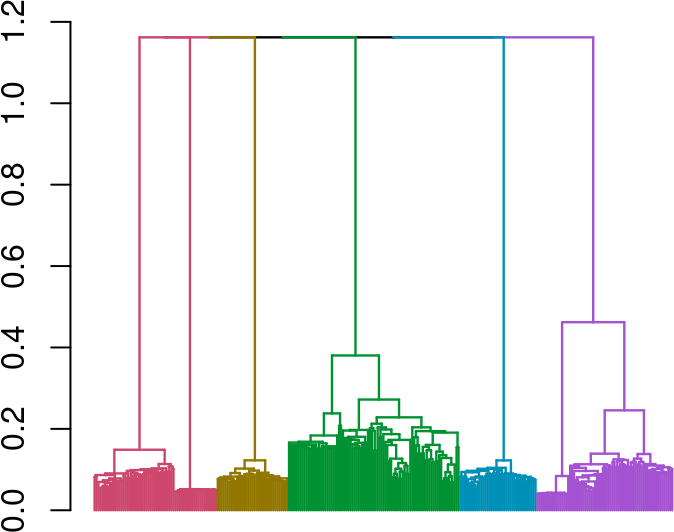##  1

We can also add a colour bar of the known label under the dendrogram as a comparison of the achieved clustering results.

Pollen.labels <- data.frame(Tissue = Pollen.Label.Tissue,
CellLine = Pollen.Label.CellLine)
HGC.PlotDendrogram(tree = Pollen.ClusteringTree,
k = 5, plot.label = TRUE,
labels = Pollen.labels)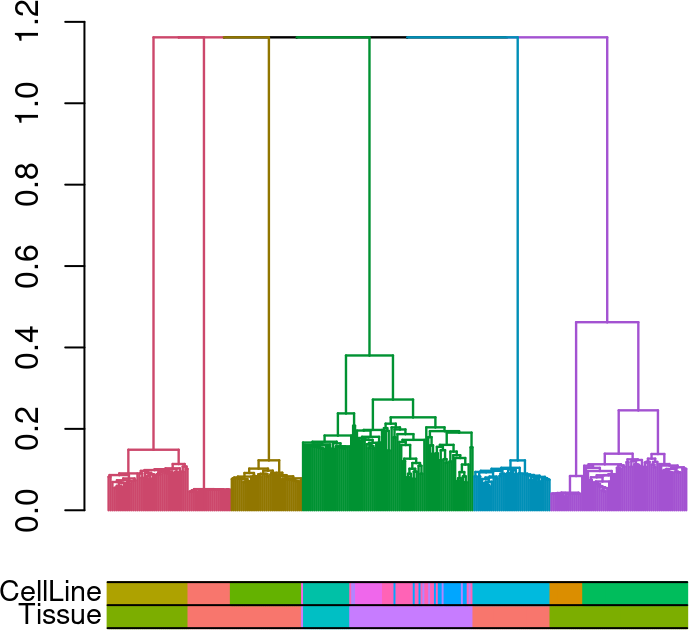##  1

3.5 Evaluation of the clustering results

For datasets with known labels, the clustering results of HGC can be evaluated by comparing the consistence between the known labels and the achieved clusters. Adjusted Rand Index (ARI) is a wildly used statistics for this purpose. Here we calculate the ARIs of the clustering results at different levels of the dendrogram with the two known labels.

ARI.mat <- HGC.PlotARIs(tree = Pollen.ClusteringTree,
labels = Pollen.labels)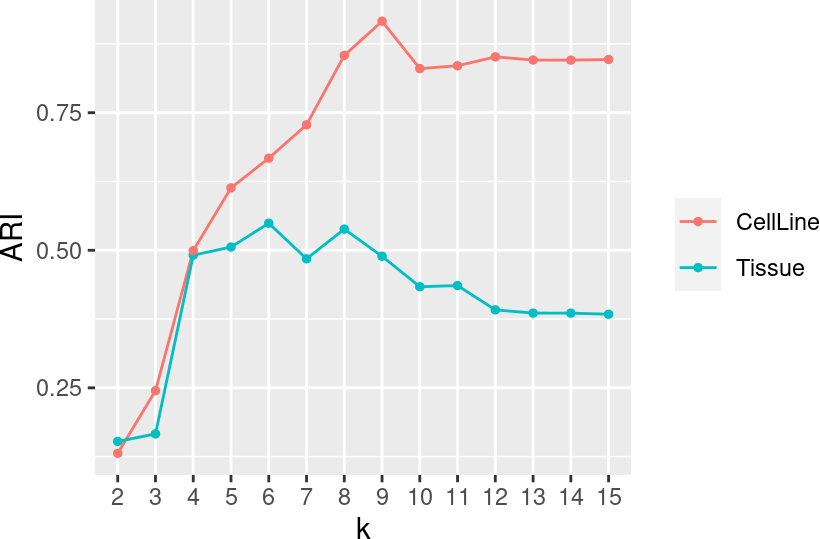4 Time complexity analysis of HGC

Our work shows that the dendrogram construction in HGC has a linear time complexity. For advanced users, HGC provides functions to conduct time complexity analysis on their own data. The construction of the dendrogram is a recursive procedure of two steps: 1. find the nearest neighbour pair, 2. merge the node pair and update the graph. For different data structures of graph, there’s a trade-off between the time consumptions of the two steps. Generally speaking, storing more information about the graph makes it faster to find the nearest neighbour pair (step 1) but slower to update the graph (step 2). We have experimented several datasets and chosen the best data structure for the overall efficiency.

The key parameters related to the time consumptions of the two steps are the length of the nearest neighbor chains and the number of nodes needed to be updated in each iteration, respectively (for more details, please refer to our preprint).HGC provides functions to record and visualize these parameters.

Pollen.ParameterRecord <- HGC.parameter(G = Pollen.SNN)

HGC.PlotParameter(Pollen.ParameterRecord, parameter = "CL")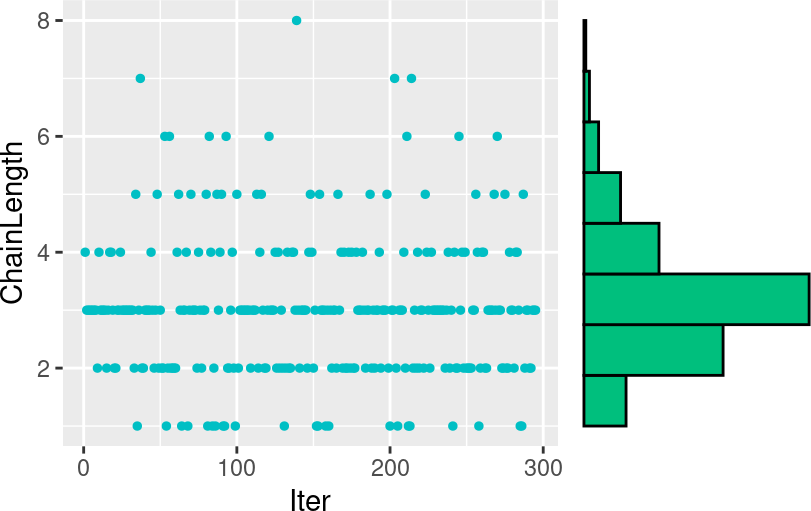##  1
HGC.PlotParameter(Pollen.ParameterRecord, parameter = "ANN")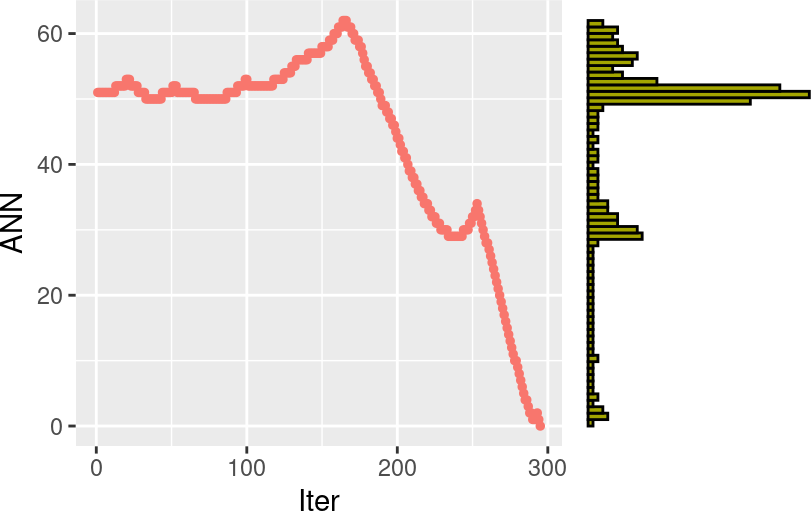##  1